# Permutations and Combinations

Permutations and Combinations
Go back to  'Data'

Sam usually takes one main course and a drink. Today he has the choice of burger, pizza, hot dog, watermelon juice and orange juice. What are all the possible combinations that he can try?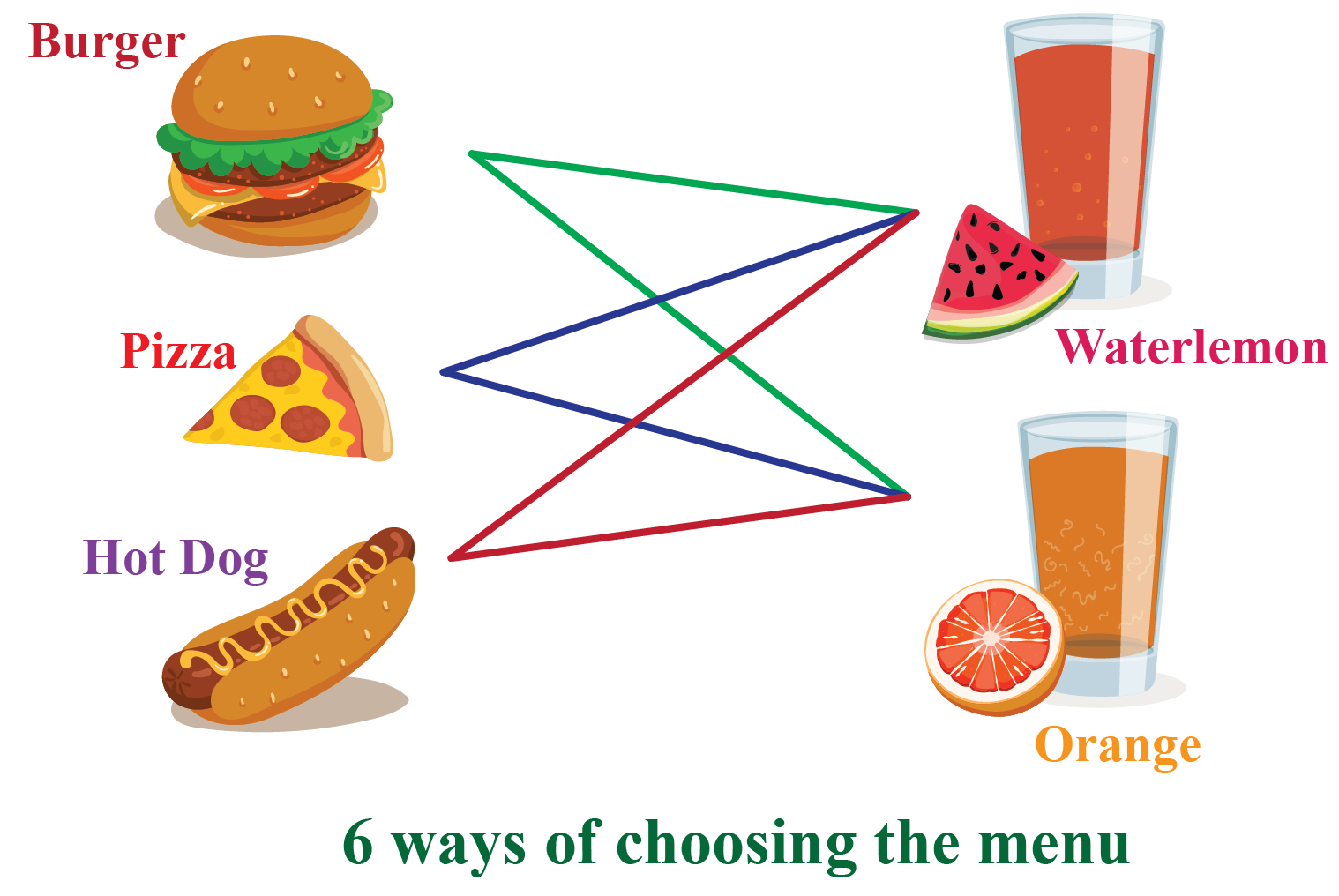There are 3 main course choices and 2 drink choices. We multiply to find the combinations. $$3\times 2=6$$.

Thus Sam can try 6 combinations.

In this min-lesson, let's learn about  permutation, arrangement, combination, collections, factorials, worksheets, formulas, examples

## Lesson Plan

 1 What Are Permutations And Combinations? 2 Tips and Tricks 3 Important Notes on Permutations And Combinations 4 Solved Examples on Permutations And Combinations 5 Challenging Questions on Permutations And Combinations 6 Interactive Questions on Permutations And Combinations

## What Are Permutations And Combinations?

The process of selecting r items out of n items is called permutations and combinations.

### Differences between permutations and combinations

Permutations Combinations
Arranging r items out of n items. Selecting r items out of n items.

There are 5 students in a group. The teacher is going to pick 3 students for a prize.

The first person she picks will get the 1st prize, the second student, the 2nd prize and 3rd prize, the third.

There are 5 students in a group. The teacher is going to pick 3 students for a prize. All get the same prize.

Solution: 60 ways Solution: 10 ways

Do you know how we got the solution?

To get on the hook of it, let's learn to find the factorials.

## Factorials

The factorial function is a product of the series of descending natural numbers.

\begin{align} n! &= 1.2.3.4.…n\\ &= \text{Product of the first n positive integers}\\&= n(n-1)(n-2)…..(3)(2)(1)\end{align}

Examples:

$$3! = 3\times 2\times 1$$

$$4! = 4\times3\times 2\times 1$$

$$4! = 4\times 3!$$

 $$n! = n \times (n - 1)!$$

We use the factorials in finding the permuations and the combinations.

## Permutations And Arrangements

A permutation is an ordered arrangement of outcomes. It is an ordered combination.

It is represented in the following ways: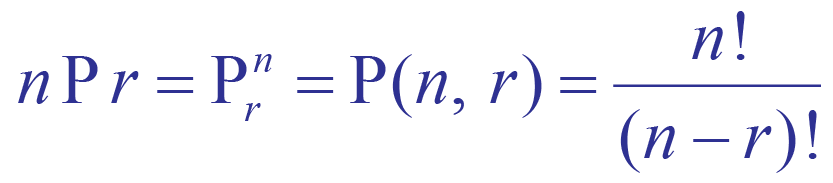Picking 3 students in order from a group of 5 to give away prizes based on their performance in athletics.

To give the first prize you pick in 5 ways.

To give the second, you pick in 4 ways.

To give the third, you pick in 3 ways.

Thus for getting the number of ways for arranging 3 persons from 5 persons, we multiply the options available to us.

We do it in 5 . 4 . 3 ways.

By analyzing this way, we find that this could be done in $$5! \times 4 ! \times 3! = 60$$ ways.

This is generalized using the formula:

 nPr = $$\dfrac{{n!}}{{(n - r)!}}$$

Examples are used to show permutation with repetition and permutation without repetition.

Example 1: There are 3 distinct letters in the word CAT.

The arrangements of the letters of that word is

$3P_3 = 3! = 6 \text{ways}$

CAT     ATC    TCA

CTA     ACT    TAC

The letters in CAT are not repeated.

Example 2: Consider the 4-letter word CUCUMBER.

U and C repeated twice.

The arrangements of the letters of that word is

$8P_8 = 8! = 40320 \text{ways}$

The arrangements of the letters C is

$2P_2 = 2! = 2 \ \text{ways}$

The arrangements of the letters U is

$2P_2 = 2! = 2 \ \text{ways}$

$$\therefore$$ We divide $$8P_8$$ and $$2P_2\times 2P_2$$ and get the final arrangement. i.e

$\dfrac{40320}{4}=10080 \text{ways}$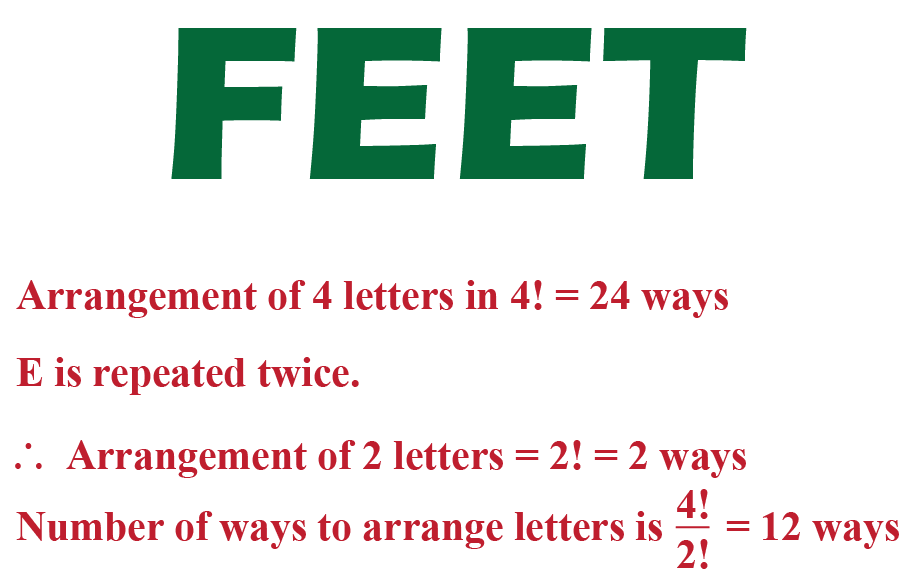Try to play with the objects in the following simulation and understand how permutations happen.

## Combinations And Collections

A combination is an arrangement of outcomes in which the order does not matter.

It is r objects chosen from n objects and the order is not important.

It is represented in the following ways: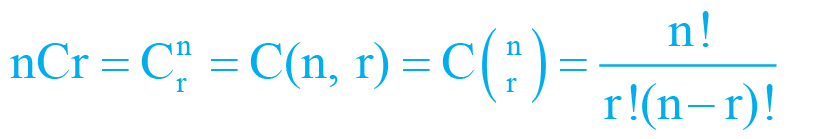Example: Select 3 books from 5 books on the shelf.

We can choose it in $$5P_3 = 60$$ ways.

The possible ways the books could be selected doesn't require an order as we can choose any at random.

Thus we divide 60 by 3 !. i.e. $$\dfrac{60}{6} = 10$$ ways.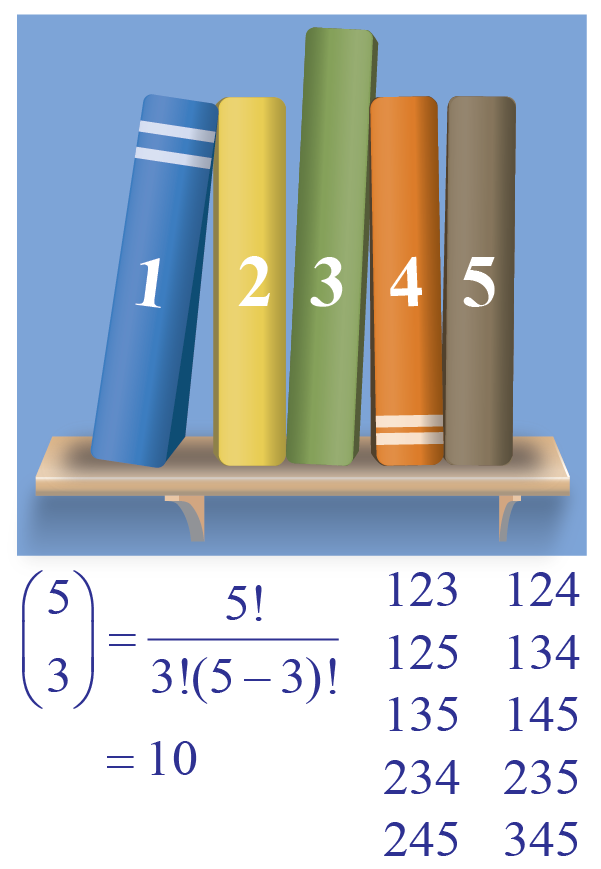nCr = $$\dfrac{{n!}}{{r!(n - r)!}}$$

Try to play with the objects in the following simulation and understand how combinations happen.

.Tips and Tricks
• When the order doesn't matter, it is a Combination.
• Hint : group, sample, selection
• When the order does matter it is a Permutation.
• Hint :arrangement, schedule, order

## Applications of Permutations And Combinations

Permutations : Allocation of phone numbers according to the country code, area codes, Assigning Car number plates according to the state codes, setting up of passwords and passcodes to maintain the security credentials.

Combinations: selecting nominees for various positions, selecting the lottery numbers.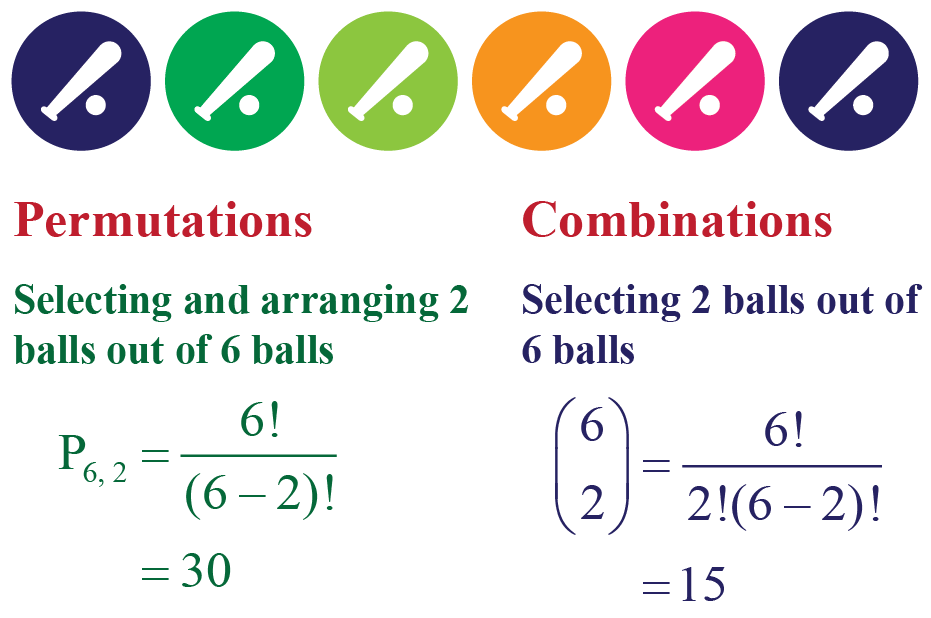More Important Topics
Numbers
Algebra
Geometry
Measurement
Money
Data
Trigonometry
Calculus